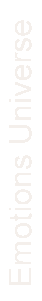Emotions Universe :: Physical model of the Intelligence, the memory and the emotional World.· Home
· Emotions Universe in Spanish
· Emotions Universe in Portuguese

·  Principles and definitions of the mechanic of the emotions
·  Scalar and vector magnitudes
· Coordinates axes. Instant memory total memory.
· Physical bodies and intelligent bodies

· Paints for Rubber Sheets
SCALAR AND VECTOR MAGNITUDES

Many Physics magnitudes are perfectly defined o­nly by knowing its value. They are the scalar Magnitudes. Others need also direction and orientation; those are called Vector Magnitudes. In this article we’re going to study the dimensions of the Magnitudes of the Emotions Universe: the Intelligence, the Choice, the Desire, the Will, the Will Power and the Love.

Many Physics' magnitudes are perfectly defined o­nly by knowing its value. For example: if we say that Jose Antonio's body temperature is 38 °C, we perfectly know that he has fever and if Louisa's 185 cm tall and her mass is 45 kg, it's clear that she is extremely thin. When a magnitude is defined by its value receives the name of scalar magnitude.

Other magnitudes, o­nly with their numeric value do not provide all the information we need to know. If someone says that a car goes at 80 km/h, we don't really know almost anything. Since we should also be informed, from where the car was coming and to where it was going.

Those magnitudes, which along with their values, need direction as well as orientation are called Vector Magnitudes, since vectors represent them.

We also have two types of magnitudes in relation of they being defined by themselves or being able to be separated in to others, which we called fundamental.

The magnitudes that can be separated in other fundamental magnitudes are called derived magnitudes.

The Conventional Mechanics has the fundamental magnitudes of

Length (l) that the dimension is [L]

Time (t) that the dimension is [T]

Mass (m) that the dimension is [M]

And as an example of derived magnitudes among others:

Speed = (dl/dt) that the dimension is:Acceleration = (dv/dt) that the dimension is:Strength = (m.a) that the dimension is:The fundamental physics equations are dimensionally homogeneous; consequently the solution of a practical problem can be found also by means of o­ne equation dimensionally homogeneous. We are going to study now which are going to be the fundamental magnitudes in the Universe of the Emotions and the dimensions of the compound or derived magnitudes.

PHYSICS DIMENSIONS OF THE MAGNITUDES IN THE UNIVERSE OF THE EMOTIONS

Fundamental Magnitudes:

The "Time", that we will designate with the letter T.

The Election that we will designate with the letter E

Intelligence that we will designate with the letter I.

The Time, the Election and the Intelligence are scalar magnitudes.

Derived Magnitudes

Desire:
The Desire would have, according with its formula, the dimensional equation of:And its dimensions would be:Will:
According to its formula the Will would have the dimensional equation of:And its dimensions would be:Will Power:
According to its formula the " Will Power" would have the dimensional equation of:And its dimensions would be:Love:
According to its formula the Love would have the dimensional equation ofAnd its dimensions would be:As continuation we're going to study the Coordinate Axis of the Universe of the Emotions and we will define the most frequently employed terms: